# Test Prep Plan - Take a practice test

Take this practice test to check your existing knowledge of the course material. We'll review your answers and create a Test Prep Plan for you based on your results.
How Test Prep Plans work
1
2Based on your results, we'll create a customized Test Prep Plan just for you!
3Study smarter
Study more effectively: skip concepts you already know and focus on what you still need to learn.

# Limits in Math: Help and Review Chapter Exam

Exam Instructions:

Choose your answers to the questions and click 'Next' to see the next set of questions. You can skip questions if you would like and come back to them later with the yellow "Go To First Skipped Question" button. When you have completed the practice exam, a green submit button will appear. Click it to see your results. Good luck!

### Page 1

#### Question 1 1. Solve for the limit: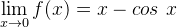#### Question 2 2. Using the given data, predict the likely value of the continuous function z.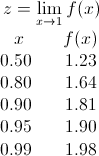#### Question 3 3. Evaluate the limit below.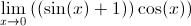#### Question 4 4. Use the graph to solve for z.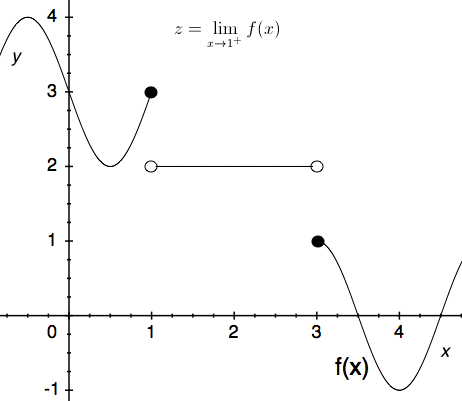#### Question 5 5. Using the graph, find the value of z, then add 1.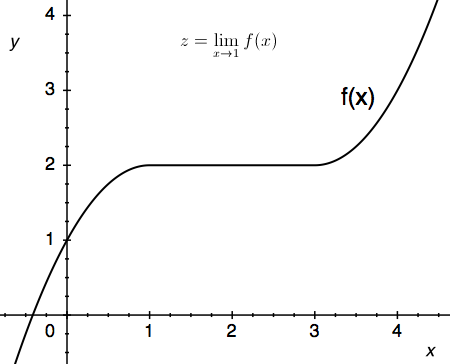### Page 2

#### Question 6 6. Using the given data, solve for the continuous function y.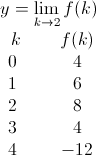#### Question 7 7. Which of the following is equivalent to the statement below?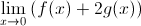#### Question 8 8. Use the given graph and formula to solve for z.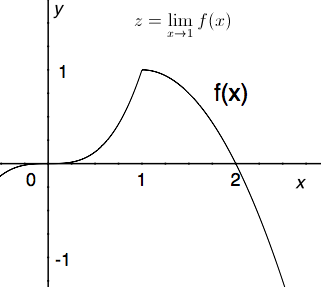#### Question 9 9. The floor function is graphically represented as a stepwise function. What is the limit of f(x) = floor(x) as x approaches 4?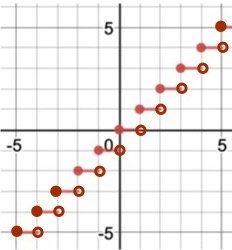#### Question 10 10. Using the squeeze theorem, what is z?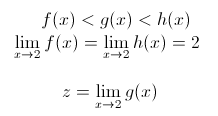### Page 3

#### Question 11 11. The expression below can also be written as: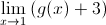#### Question 13 13. Evaluate the limit below.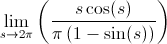#### Question 14 14. Use the graph to solve for z.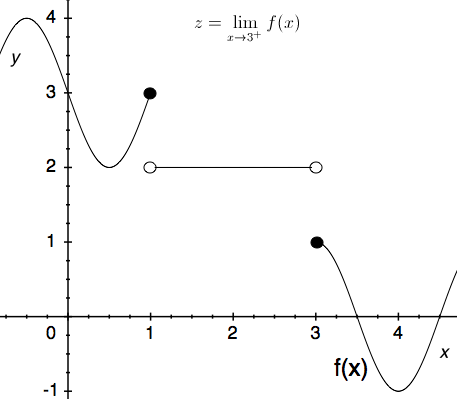#### Question 15 15. Which of the following is true?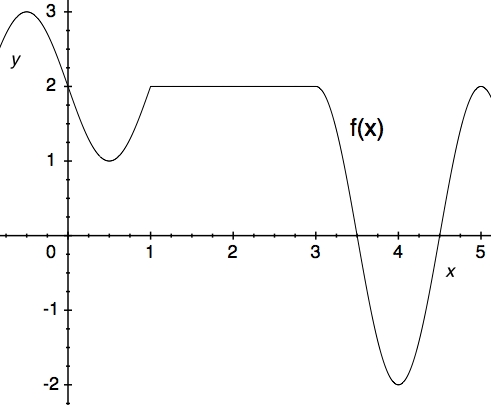### Page 4

#### Question 16 16. Use the graph to solve for z.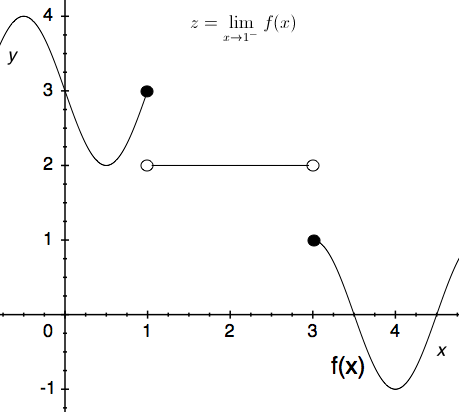#### Question 17 17. The expression below can also be written as: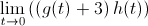#### Question 18 18. Use the graph to solve for z.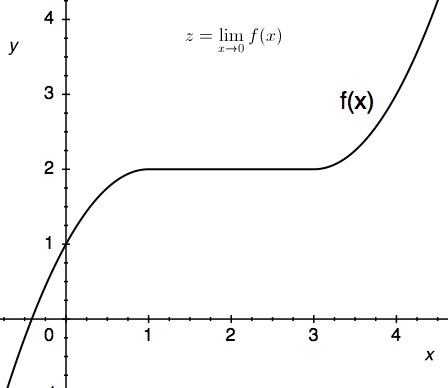#### Question 20 20. Using the squeeze theorem, what is z?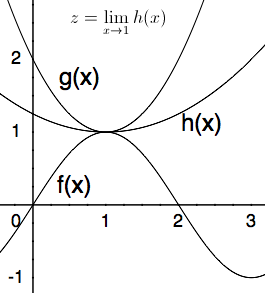### Page 5

#### Question 22 22. The ceiling function is graphically translated as a stepwise function. What is the limit of f(x) = ceil(x) as x approaches 4.5?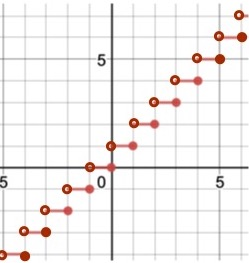#### Question 25 25. Using the squeeze theorem, what is z?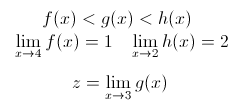### Page 6

#### Question 26 26. Use the given formula to solve for z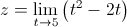#### Question 27 27. Calculate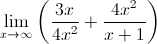#### Question 28 28. Calculate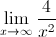#### Question 29 29. Using the squeeze theorem, what is z?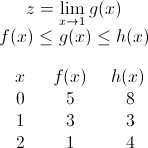#### Question 30 30. Use the given graph and formula to solve for z.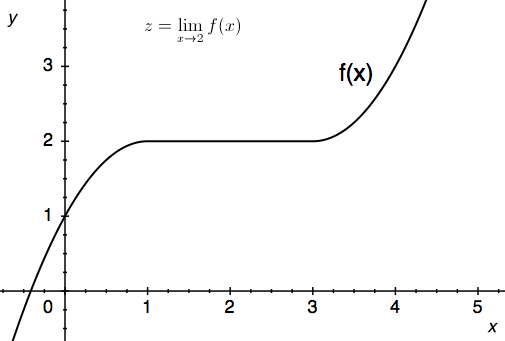#### Limits in Math: Help and Review Chapter Exam Instructions

Choose your answers to the questions and click 'Next' to see the next set of questions. You can skip questions if you would like and come back to them later with the yellow "Go To First Skipped Question" button. When you have completed the practice exam, a green submit button will appear. Click it to see your results. Good luck!

Support